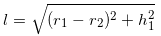Newbie

# An oil funnel made of tin sheet consists of a 10 cm long cylindrical portion attached to a frustum of a cone. If the total height is 22 cm, diameter of the cylindrical portion is 8 cm and the diameter of the top of the funnel is 18 cm, find the area of the tin sheet required to make the funnel (see Fig.). Q.5

• 0

Give me the easiest and simple solution of the chapter surface areas and volumes  of ncert class 10th . Find the simple and easiest solution of the exercise 13.5 question number 5 give me the best solution of this question.An oil funnel made of tin sheet consists of a 10 cm long cylindrical portion attached to a frustum of a cone. If the total height is 22 cm, diameter of the cylindrical portion is 8 cm and the diameter of the top of the funnel is 18 cm, find the area of the tin sheet required to make the funnel (see Fig.).

Share

1. Given,

Diameter of upper circular end of frustum part = 18 cm

So, radius (r1) = 9 cm

Now, the radius of the lower circular end of frustum (r2) will be equal to the radius of the circular end of the cylinder

So, r2 = 8/2 = 4 cm

Now, height (h1) of the frustum section = 22 – 10 = 12 cm

And,

Height (h2) of cylindrical section = 10 cm (given)

Now, the slant height will be-Or, l = 13 cm

Area of tin sheet required = CSA of frustum part + CSA of cylindrical part

= π(r1+r2)l+2πr2h2

Solving this we get,

Area of tin sheet required = 782×(4/7) cm2

• 0